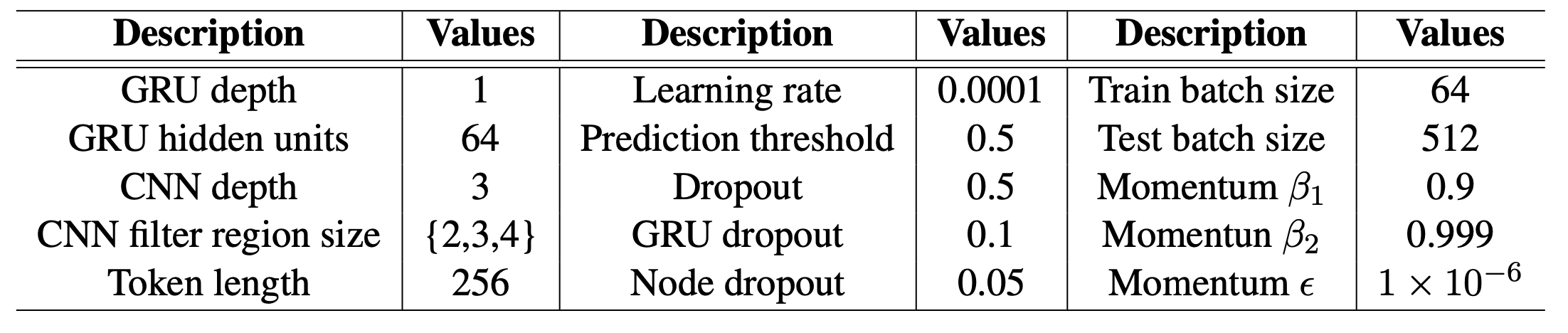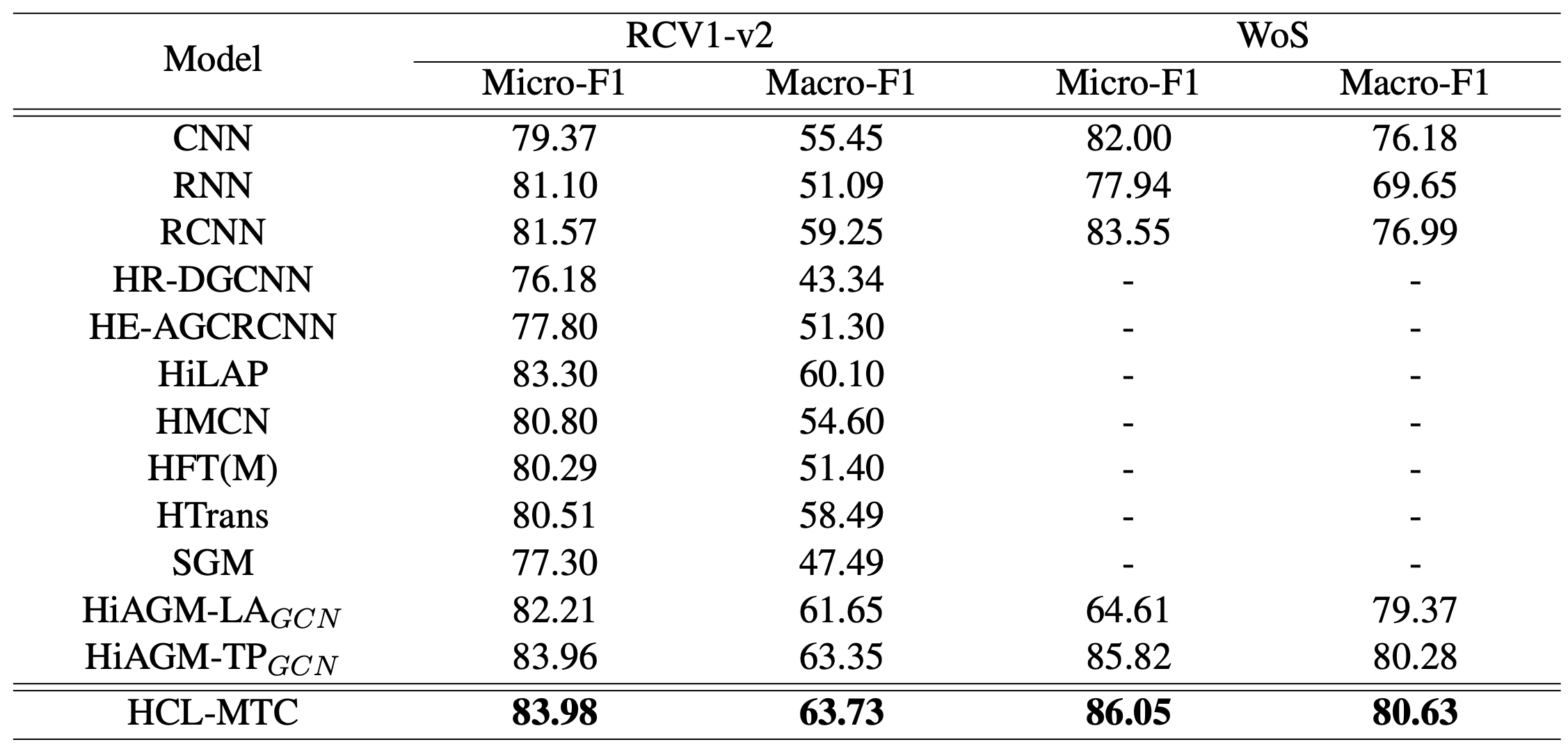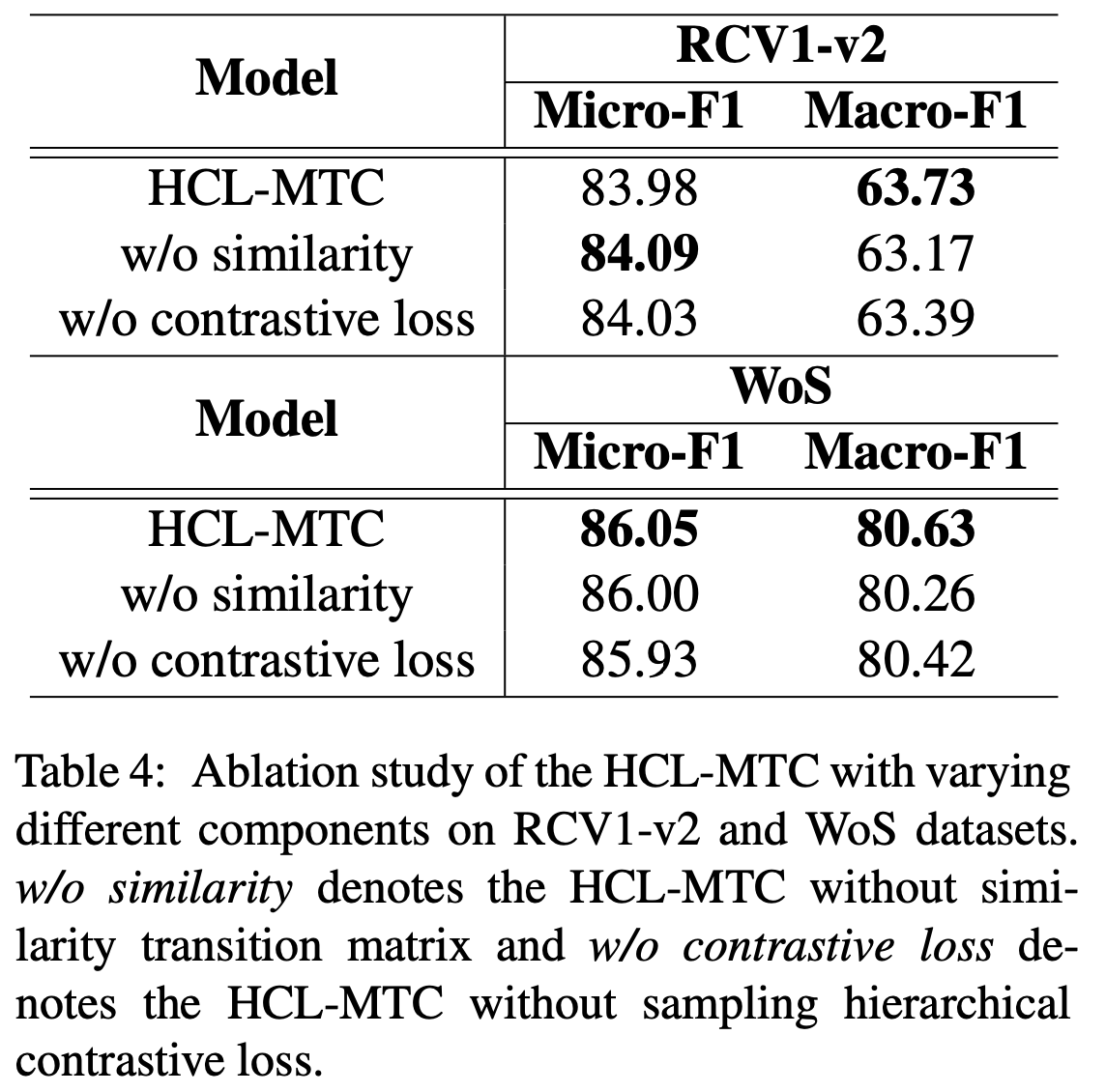ACL ARR 2022，提出层次对比学习，学习标签间的区别信息。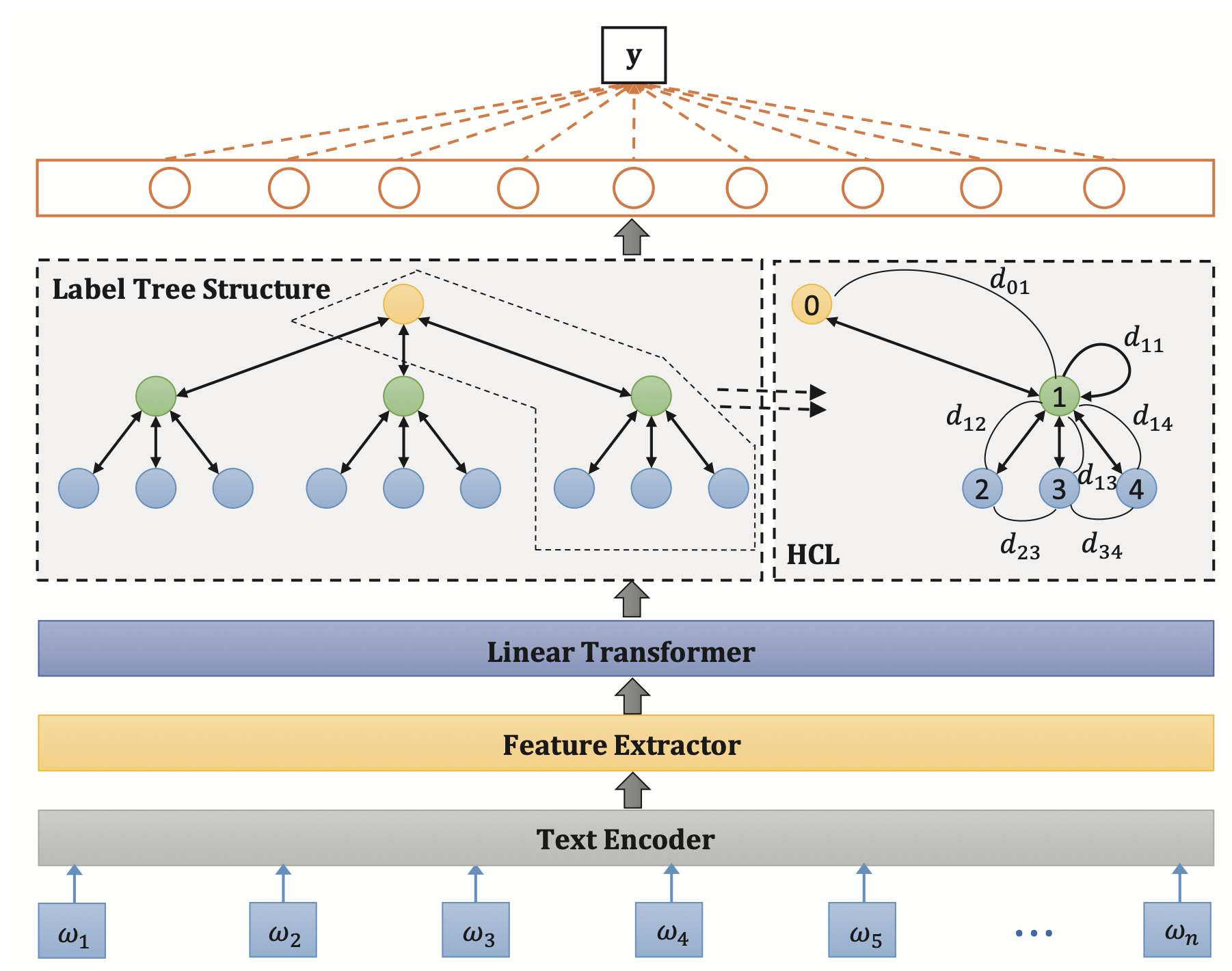## Background

MLTC任务可以分为两种方法：直接从文本信息预测以及从文本标签的混合信息中预测。前者忽略了标签间的信息，后者可以学习标签的层次信息。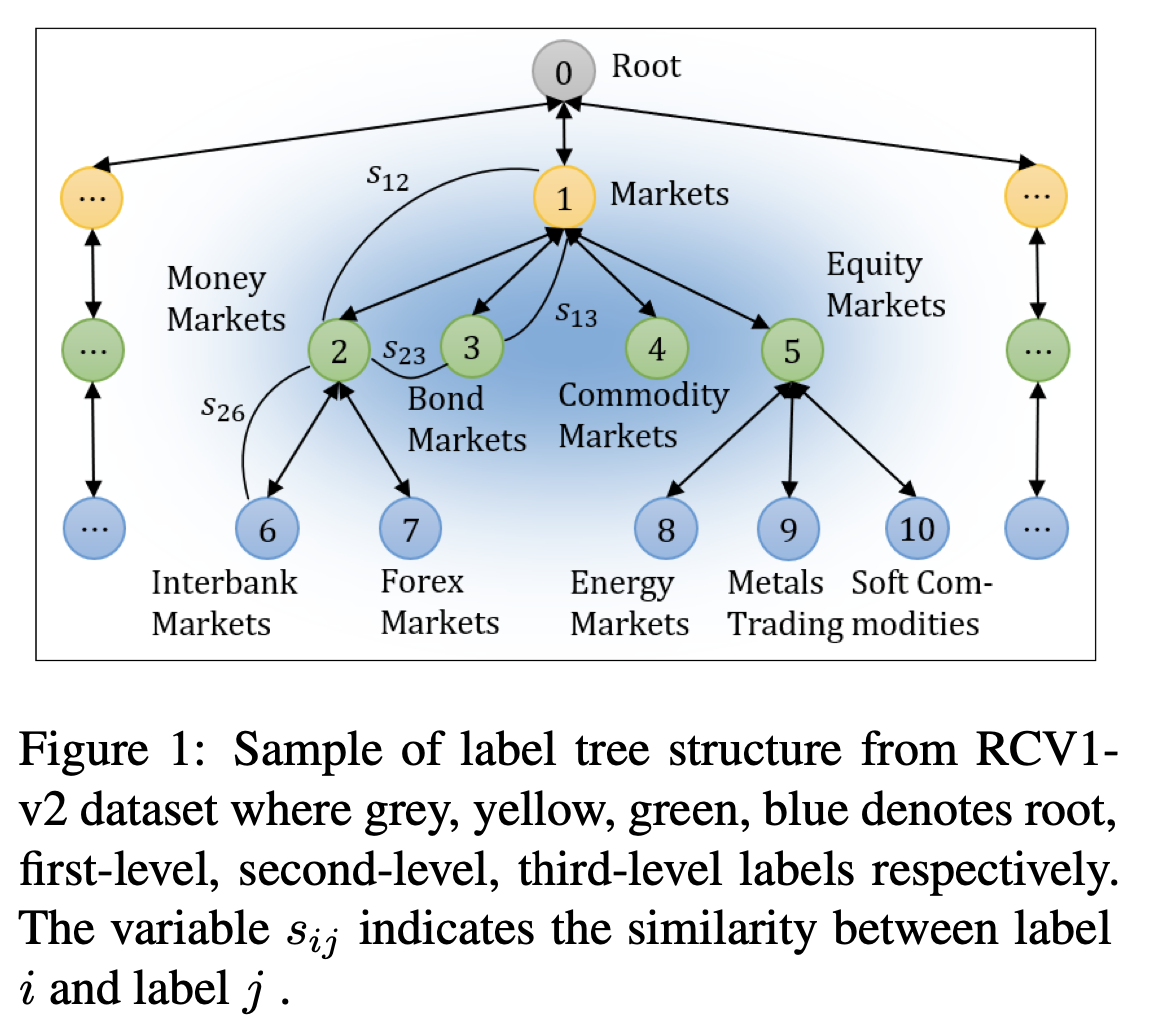## Method

Recursive Regularization for Large-scale Classification with Hierarchical and Graphical Dependencies, KDD 2013 [paper]

\begin{align} L_c &= -\sum_{i=1}^m[y_i\log(y_i')+(1-y_i)\log(1-y_i')] \\ L_r &= \sum_{i\in\mathcal{V}}\sum_{j\in child(i)}\frac12\lVert w_i-w_j\rVert^2 \\ L &= L_c + \lambda_1L_r + \lambda_2L_d \end{align}

## Experiment